CAT 2016 Quant sample question bank - Inequality : Ascent Education - MBA CAT Preparation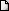LATEST NEWS • Ascent Success Stories • TANCET 16 Classes @ Chennai • XAT 16 Classes @ Chennai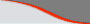Home | TANCET Coaching Class | TANCET study material | Online TANCET Course | Contact us
 Ascent's Courses TANCET Coaching Chennai Online TANCET Course TANCET MBA Study Material CAT Classroom Chennai CAT Correspondence India Mock CAT Series GRE Coaching Chennai GMAT Coaching Chennai Online GMAT Course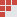US B Schools Faculty List Jobs @ Ascent Testimonials Ascent Success Stories Contact Us +91 44 4500 8484 +91 96000 48484
You are here: Home » CAT Questions » Inequalities » Domains and Ranges

#### Inequality - Math Questions & Answers - CAT 2016

Question 4 the day : April 01, 2005

The Math practice question for the day is an inequality question. Inequality is one of the easiest set of questions if the basic concepts are well understood.

Question
For what range of values of 'x' will the inequality 15x -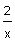> 1?

(1) x > 0.4
(2) x <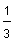(3) -< x < 0.4, x >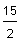(4) -< x < 0, x >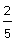Correct choice (4). Correct Answer - (-< x < 0, x >)

We can rewrite the above inequality as 15x -- 1 > 0
i.e.,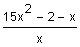> 0
or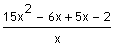> 0.
Factorizing we get,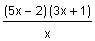> 0
The above inequality will hold good if the numerator and denominator are both positive or are both negative.

Case 1: When (5x - 2)(3x + 1) > 0 and x > 0
This will hold true for values of 'x' that do not lie between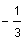andand for x > 0.
Combining all these conditions, we get x >Case 2: When (5x - 2)(3x + 1) < 0 and x < 0
This will hold true for the following values of 'x':< x <and x < 0.
Combining, we get< x < 0.

Therefore, the final range for which the above inequality will hold true is given by< x < 0 and x >.

## XLRI XAT TANCET Practice Question Samples

TANCET Questionbank
 TANCET MBA MCA Practice Questions with detailed explanation and shortcuts.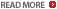GMAT Math Books
 Comprehensive study material for GMAT Quant. 1800+ questions.CAT Online Course

 GMAT Diagnostic Test

 CAT Discussion Forum

 GMAT Math Lesson Book | SAT prep | GRE Classes @ Chennai | GMAT Coaching @ Chennai | TANCET Mailing List © 2002 - 17 ASCENT Education all rights reserved. Terms & Condition | Privacy Policy